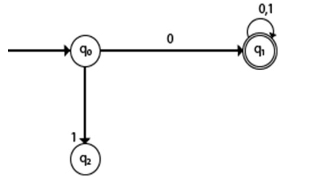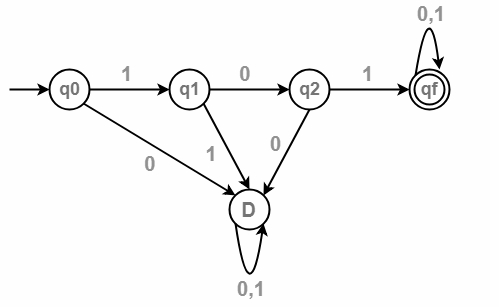# Construct DFA with Σ= {0,1} accepts all strings with 0.

A Deterministic Finite automata (DFA) is a collection of defined as a 5-tuples and is as follows −

M=(Q, Σ, δ,q0,F)

Where,

• Q: Finite set called states.
• Σ: Finite set called alphabets.
• δ: Q × Σ → Q is the transition function.
• q0 ∈ Q is the start or initial state.
• F: Final or accept state.

## Example 1

The DFA accepts all strings starting with 0

The language L= {0,01,001,010,0010,000101,…}

## Transition diagram

The transition diagram is as follows −## Explanation

• Step 1 − q0 is the initial state on input ‘0’ it goes to q1, which is the final state, and ‘0’ string is accepted.
• Step 2 − q0 on ‘1’ goes to q2 which is dead state because for q2 there is no path to reach to the final state.
• Step 3 − q1 on input ‘0’ and ‘1’ goes to q1 itself which is the final state.

## Transition table

The transition table is as follows −

State/input symbol01
->q0q1q2
q1q1q1
q2--

## Example 2

Construct DFA for the language accepting strings starting with ‘101’

• Then the length of the substring = 3.

Therefore, Minimum number of states in the DFA = 3 + 2 = 5.

The minimized DFA has five states.

The language L= {101,1011,10110,101101,.........}

The transition diagram is as follows −## Explanation

• Step 1 − q0 is an initial state on input ‘1’ goes to q1 and on input ‘0’ leads to a dead state.
• Step 2 − q1 on input ‘0’ goes to q2 and on ‘1’ goes to dead state.
• Step 3 − q2 on input ‘1’ goes to qf which is the final state, and on ‘0’ goes to dead state.
• Step 4 − qf is the final state, on input ‘1’ and ‘0’ it goes to qf itself.